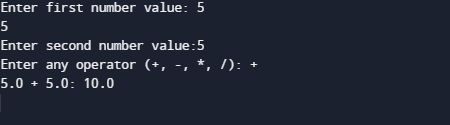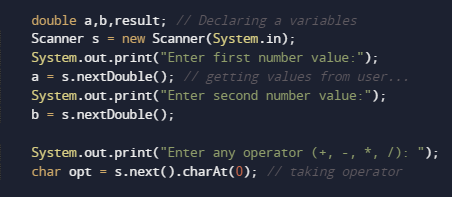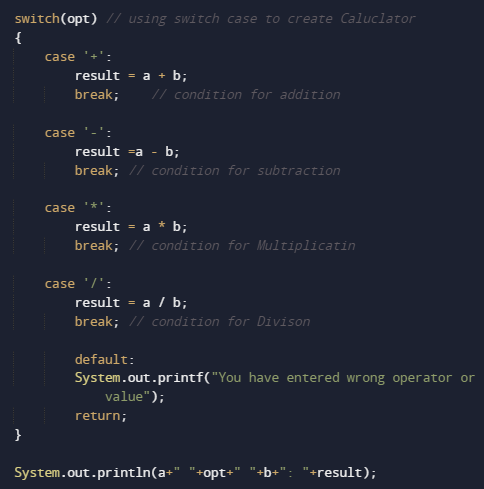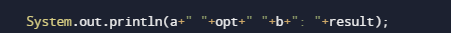# Create Simple Calculator Program In Java

In this tutorial you will learn about the Create Simple Calculator Program In Java and its application with practical example.

In this tutorial, we will learn to create a Java program to make a simple Calculator using Switch case using Java programming.

## Prerequisites

Before starting with this tutorial we assume that you are best aware of the following Java programming topics:

• Java Operators.
• Basic Input and Output
• Class and Object.
• Basic Java programming.
• if-else statements.
• Switch case.
• Nested if-else Statements.
• Java Scanner class.

## What is calculator?

Calculator is a Device that is used to performing mathematical operation like arithmetic operations on numbers.With the Calculators we can perform operation like Addition, Subtraction,Multiplication, and Division… and function like that.

## Create Simple Calculator Program In Java

In this our program we will create a small calculator using switch case . We would first declared and initialized the required variables. Next, we would prompt user to input the two values . Later in program we will ask operator and perform that particular operation in our program.

## OutputIn the above program, we have first declared and initialized a set variables required in the program.

• a= it will hold First value.
• b= it will hold second value.
• opt=it will hold operator value.

After declaring variables  in the next statement user will be prompted to enter two values and  which will be assigned to variable ‘a‘ and ‘b‘ respectively as shown in image below.Afterword we will ask user to input symbol to perform following task like( +,-,*,/) to perform following task.After taking values opt we will pass the value of options to switch case to Calculate resultThe above  statements compute the addition of two numbers and print the output.and finally we will print the result.

In this tutorial we have learn about the Create Simple Calculator Program In Java and its application with practical example. I hope you will like this tutorial.